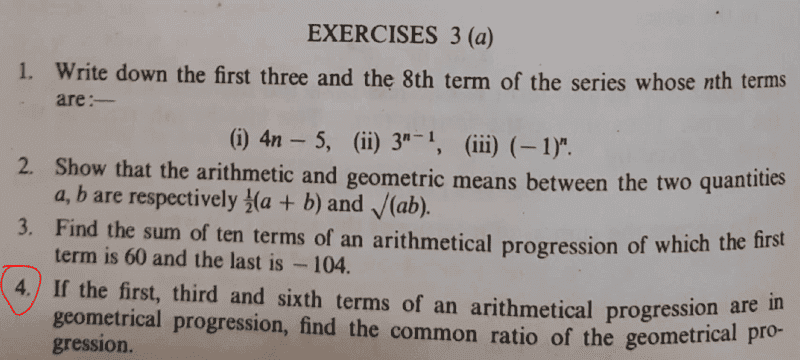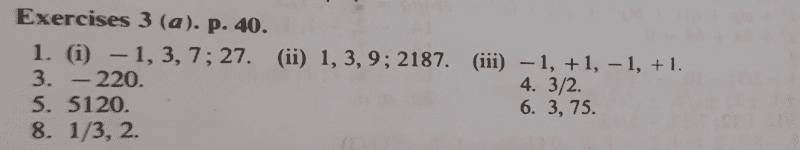# Find the common ratio of a geometric progression for this arithmetic sequence

Gold Member
Homework Statement:
If the first, third and sixth term of an arithmetical progression are in geometrical progression, find the common ratio of the geometrical progression.
Relevant Equations:
geometric mean
My attempt;
The terms in the arithmetic sequence are ;##[ a, a+2d,a+5d]##.
It follows that;
Common ratio ##r=\dfrac{a+2d}{a}=\dfrac{a+5d}{a+2d}##
##⇒ar+2rd=a+2d+3d##
##ar+2rd=ar+3d##
##ar+2rd-ar=3d##
##2rd=3d##
##r=\dfrac{3d}{2d}=\dfrac{3}{2}##

The solution given on the textbook is ##r=\dfrac{3}{2}##. Seeking alternative method guys.

Last edited:

Mentor
Homework Statement:: If the first, third and sixth term of an arithmetical progression are in geometrical progression, find the common ratio of the geometrical progression.
Relevant Equations:: geometric mean

My attempt;
The terms in the arithmetic sequence are ;##[ a, a+2d,a+5d]##.
It follows that;
Common ratio ##r=\dfrac{a+2d}{a}=\dfrac{a+5d}{a+2d}##
##⇒ar+2rd=a+2d+3d##
##ar+2rd=ar+3d##
##ar+2rd-ar=3d##
##2rd=3d##
##r=\dfrac{3d}{2d}=\dfrac{3}{2}##

The solution given on the textbook is ##r=\dfrac{3}{2}##.
chwala said:
Seeking alternative method guys.
Why? The approach you wrote is logical and consistent with the given information. The only change I would make would be in the line that starts with "The terms in the arithmetic sequence are ..."
Those are the first, third, and sixth terms of the arithmetic sequence.

A question for you, though, is this: What would you do differently if you weren't given the answer to this problem?

Prof B
Homework Statement:: If the first, third and sixth term of an arithmetical progression are in geometrical progression, find the common ratio of the geometrical progression.
Relevant Equations:: geometric mean

My attempt;
The terms in the arithmetic sequence are ;##[ a, a+2d,a+5d]##.
It follows that;
Common ratio ##r=\dfrac{a+2d}{a}=\dfrac{a+5d}{a+2d}##
##⇒ar+2rd=a+2d+3d##
##ar+2rd=ar+3d##
##ar+2rd-ar=3d##
##2rd=3d##
##r=\dfrac{3d}{2d}=\dfrac{3}{2}##

The solution given on the textbook is ##r=\dfrac{3}{2}##. Seeking alternative method guys.
Your textbook is wrong. If a=0 then the sequence is 0,0,0,0,0,0 and the common ratio is undefined. If a is not 0, then the common ratio is either 1 or 3/2. You missed the r=1 solution because you divided. Instead, multiply, using the fact that each term in a geometric sequence is equal to the product of the adjacent terms.

Mentor
Your textbook is wrong. If a=0 then the sequence is 0,0,0,0,0,0 and the common ratio is undefined. If a is not 0, then the common ratio is either 1 or 3/2. You missed the r=1 solution because you divided. Instead, multiply, using the fact that each term in a geometric sequence is equal to the product of the adjacent terms.
First, a is the starting value of the sequence. If a = 0, then the arithmetic sequence is {0, d, 2d, 3d, ...}. This sequence is the zero sequence only if the common difference d happens also to be 0.

Second, each term in a geometric sequence is equal to the product of the common ratio and the previous term, not the product of the adjacent terms.

Finally, from the equation ##r = \frac{a + 2d}a##, and assuming ##a \ne 0##, we get ##ra = a + 2d## or ##a(r - 1) = 2d##. If r = 1 as you said, then d = 0 as well, making the arithmetic sequence {a, a, a, ...}, not a very interesting arithmetic or geometric sequence. Perhaps the textbook had additional constraints that weren't included in the problem statement here.

Gold Member
Why? The approach you wrote is logical and consistent with the given information. The only change I would make would be in the line that starts with "The terms in the arithmetic sequence are ..."
Those are the first, third, and sixth terms of the arithmetic sequence.

A question for you, though, is this: What would you do differently if you weren't given the answer to this problem?
I may not have an immediate answer @Mark44 ...the purpose of me sharing the questions is to enjoy the beauty of maths with the viewers, more so getting diverse opinion on a subject matter...I guess I mentioned that in the past.

Gold Member
First, a is the starting value of the sequence. If a = 0, then the arithmetic sequence is {0, d, 2d, 3d, ...}. This sequence is the zero sequence only if the common difference d happens also to be 0.

Second, each term in a geometric sequence is equal to the product of the common ratio and the previous term, not the product of the adjacent terms.

Finally, from the equation ##r = \frac{a + 2d}a##, and assuming ##a \ne 0##, we get ##ra = a + 2d## or ##a(r - 1) = 2d##. If r = 1 as you said, then d = 0 as well, making the arithmetic sequence {a, a, a, ...}, not a very interesting arithmetic or geometric sequence. Perhaps the textbook had additional constraints that weren't included in the problem statement here.
The textbook has no additional constraints...the problem set here is word- word as it is on the text.Gold Member
Your textbook is wrong. If a=0 then the sequence is 0,0,0,0,0,0 and the common ratio is undefined. If a is not 0, then the common ratio is either 1 or 3/2. You missed the r=1 solution because you divided. Instead, multiply, using the fact that each term in a geometric sequence is equal to the product of the adjacent terms.

@Prof B Is the set ##[{0,0,0,0,0}]## a sequence? If indeed it is, then what is the first term? common difference? is it an Arithmetic or Geometric sequence? or what type of sequence is it? and secondly,

how are you arriving at common ratio ; ##r=1?##

For starters; just to consider a geometric sequence as an example, one condition is that the common ratio ##r≠0##.

Last edited:
Mentor
@Prof B Is the set [0,0,0,0,0] a sequence? If indeed it is, then what is the first term?
Not directed to me, but I'll answer anyway. Yes, {0, 0, 0, ...} is a sequence. Its first term is 0.
common difference?
d = 0.
is it an Arithmetic or Geometric sequence? or what type of sequence is it?
It's trivially an arithmetic sequence AKA arithmetic progression. Per wikipedia's definition, https://en.wikipedia.org/wiki/Geometric_progression, the zero sequence, (with emphasis added)
In mathematics, a geometric progression, also known as a geometric sequence, is a sequence of non-zero numbers where each term after the first is found by multiplying the previous one by a fixed, non-zero number called the common ratio.
and secondly,

how are you arriving at common ratio ; r=1?
If we accept wikipedia's definition, the zero sequence isn't a geometric sequence, so the concept of common ratio doesn't apply.

•chwala
Prof B
If we accept wikipedia's definition, the zero sequence isn't a geometric sequence, so the concept of common ratio doesn't apply.
Wikipedia is fairly reliable, but not 100%. The OP should follow whatever definition is in his textbook. The textbook I teach from places no restrictions on a and r.

Prof B
Second, each term in a geometric sequence is equal to the product of the common ratio and the previous term, not the product of the adjacent terms.
Oh, thanks for catching my mistake. The square of each term is the product of the adjacent terms. The equation I solved to find all sequences with the stated property is (a+2d)(a+2d) = a(a+5d).

Prof B
how are you arriving at common ratio ; ##r=1?##
By solving the equation (a+2d)(a+2d) = a(a+5d) and then checking my answer. Give it a try. It's not hard. The solutions are d=0 and d=a/4. To avoid triggering people, let's assume a is not 0. When d=0 all the terms in the sequence are equal so r=1. When d=0.25a, the third term is 1.5a and the sixth term is 2.25a so the common ratio is 1.5.

Last edited:
Gold Member
By solving the equation (a+2d)(a+2d) = a(a+5d) and then checking my answer. Give it a try. It's not hard. The solutions are d=0 and d=a/4. To avoid triggering people, let's assume a is not 0. When d=0 all the terms in the sequence are equal so r=1. When d=0.25a, the third term is 1.5a and the sixth term is 2.25a so the common ratio is 1.5.
Why should we assume? Not getting it...

Gold Member
Wikipedia is fairly reliable, but not 100%. The OP should follow whatever definition is in his textbook. The textbook I teach from places no restrictions on a and r.
Which textbook is this?

Gold Member
Wikipedia is fairly reliable, but not 100%. The OP should follow whatever definition is in his textbook. The textbook I teach from places no restrictions on a and r.
In Mathematics, we don't follow 'whatever definition' as you want to insuniate! There has to be some reference to any given rule or concept! ...